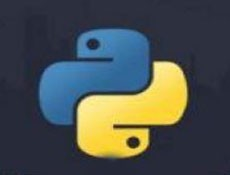# python3.6print怎么用• silencement   2019-11-07 14:13:42399```x = 1
y = 2
z = 3
print("the values are", x, y, z)
#打印结果为 the values are 1 2 3```

```x = 1
y = 2
z = 3
print("the values are %d %7.5f %s" % (x, y, z))```

```f = open("file_path", 'w')
print >>f, "hello world"  #这是python2中的用法，在python3的具体方法请看下文
...
f.close()```

print()函数

Python3中最重要的更改之一就是将print转变为函数，在python2中他是一个关键字

```print("the values are",x, y, z, end = '') #禁止换行符
print("the values are",x, y, z, file = f) #重定向到文件对象f
print("the values are",x, y, z, sep = ',') #在值之间添加逗号```

python学习网，免费的python学习网站，欢迎在线学习！